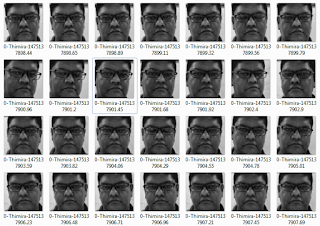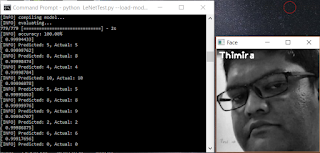# Getting the LeNet model working with Face Recognition

In my last post, I talked about how the LeNet Convolutional Neural Network model is capable of handling much more complex data than the intended MNIST dataset. We saw how it got ~99% accuracy when it learned to identify 10 faces from the raw pixel intensities.

So, let’s see the code I used to get it working.

First of all, I needed a training dataset. For that, I created a set of face images of 10 subjects with around 500 images each.The training dataset (yep, that's my face)

I use a file naming convention as <subject_label>-<subject_name>-<unique_number>.jpg (e.g. 0-Thimira-1475137898.65.jpg) for the training images to make it easier to read in and get the metadata of the images in one go. (I will do a separate post on how to easily create training datasets of face images like this).

We'll mainly be using Keras to build the model, and scikit-learn for some utility functions. We’ll need to import the following packages,
`````` from sklearn.cross_validation import train_test_split
from keras.optimizers import SGD
from keras.utils import np_utils
import numpy as np
import argparse
import cv2
import os
import sys
from PIL import Image
``````

We’ll be using the same LeNet model as the one used for the MNIST dataset,
`````` # import the necessary packages
from keras.models import Sequential
from keras.layers.convolutional import Convolution2D
from keras.layers.convolutional import MaxPooling2D
from keras.layers.core import Activation
from keras.layers.core import Flatten
from keras.layers.core import Dense

class LeNet:

@staticmethod
def build(width, height, depth, classes, weightsPath=None):
# initialize the model
model = Sequential()

# first set of CONV => RELU => POOL
input_shape=(depth, height, width)))

# second set of CONV => RELU => POOL

# set of FC => RELU layers

# softmax classifier

# if a weights path is supplied (inicating that the model was
# pre-trained), then load the weights
if weightsPath is not None:

# return the constructed network architecture
return model
``````

I use the following code to read in the images and create lists for the image data, the labels, and the label-name mappings,
`````` # Append all the absolute image paths in a list image_paths
image_paths = [os.path.join(path, f) for f in os.listdir(path)]
# images list will contain face image data. i.e. pixel intensities
images = []
# labels list will contain the label that is assigned to the image
labels = []
# dictionary/map with label to subject name
name_map = {}

for image_path in image_paths:
# Read the image and convert to grayscale
image_pil = Image.open(image_path).convert('L')
# Convert the image format into numpy array
image = np.array(image_pil, 'uint8')

# Get the label of the image
nbr = int(os.path.split(image_path).split("-"))
# Get the subject name for the label
name = os.path.split(image_path).split("-")
name_map[nbr] = name

images.append(image)
labels.append(nbr)
``````

We’ll use the train_test_split method to split the training dataset to train and test sets. I used 10% of data as the test set.
`````` (trainData, testData, trainLabels, testLabels) = train_test_split(images, labels, test_size=0.10)
``````

We need to convert the labels form a numerical value to a categorical vector. E.g. the label 3 should be converted to [0, 0, 0, 1, 0, 0, 0, 0, 0, 0]. We use the np_utils.to_categorical method for that. The value 10 is the number of classes in the dataset – 10 faces in our data.
`````` trainLabels = np_utils.to_categorical(trainLabels, 10)
testLabels = np_utils.to_categorical(testLabels, 10)
``````

Then, we need to transform our training and test data to a format that can be consumed by Keras model,
`````` trainData = np.asarray(trainData)
testData = np.asarray(testData)

trainData = trainData[:, np.newaxis, :, :] / 255.0
testData = testData[:, np.newaxis, :, :] / 255.0
``````

Keras expects the input data to be a numpy array with the shape of (samples, channels, rows, cols). When we convert the image list to a numpy array – using np.asarray in above code – we get the shape (samples, rows, cols). We add in the channels dimension as a new axis - [:, np.newaxis, :, :] – since we only have 1 dimension (since our images are grayscale). The pixel values in the images range from 0 to 255. So finally, we make the pixel intensities to a value between 0 and 1 by dividing the whole array by 255.

With our data ready, we build, compile, fit, and evaluate the LeNet model. The width and height should be the width and height of your images.
`````` opt = SGD(lr=0.01)

model = LeNet.build(width=244, height=244, depth=1, classes=10, weightsPath=args["weights"] if args["load_model"] > 0 else None)

model.compile(loss="categorical_crossentropy", optimizer=opt, metrics=["accuracy"])

model.fit(trainData, trainLabels, batch_size=128, nb_epoch=20, verbose=1)

(loss, accuracy) = model.evaluate(testData, testLabels, batch_size=128, verbose=1)
print("[INFO] accuracy: {:.2f}%".format(accuracy * 100))
``````

We’ll be getting about 99% accuracy.Accuracy of LeNet on our test dataset

Finally, we’ll randomly pick some items from the test data, and see how the model predicts them,
`````` for i in np.random.choice(np.arange(0, len(testLabels)), size=(10, )):
# classify the face
probs = model.predict(testData[np.newaxis, i])

print probs

prediction = probs.argmax(axis=1)
print probs[prediction]

image = (testData[i] * 255).astype("uint8")

name = "Subject " + str(prediction)
if prediction in name_map:
name = name_map[prediction]

cv2.putText(image, name, (5, 20), cv2.FONT_HERSHEY_PLAIN, 1.3, (255, 255, 255), 2)

# show the image and prediction
print("[INFO] Predicted: {}, Actual: {}".format(
prediction, np.argmax(testLabels[i])))
cv2.imshow("Face", image)
cv2.waitKey(0)
``````

Here you see LeNet has successfully recognized my face.LeNet recognizing my face
It works with different poses of the face too.LeNet successfully recognizing different face poses

I'm planning to see whether we can use this on a real-time face recognition task also. Will keep you posted.

Related posts:
Can the LeNet model handle Face Recognition?

Build Deeper: Deep Learning Beginners' Guide is the ultimate guide for anyone taking their first step into Deep Learning.

1.I see that you have applied LeNet model which was used on MNIST dataset for classification to perform face recognition. So what do you think is the fundamental difference between the problem of "classification" and "recognition"?

1.Hi Kushal,

That's an interesting question.

If we take the terms 'classification' and 'recognition' generally (out of the context of 'face recognition'), then we can say the following:
Classification is when we group a set of items in to two or more categories, typically done via supervised learning using a labeled training set.
Recognition is when we attempt to identify general patters among our items set, typically done via unsupervised learning and without a training set.

However, when we come back into the context of 'Face Recognition' the terms are used out of their general meaning.

When we talk about Face Recognition, what we are actually doing is classification. (and all implementations of face recognition that I'm aware of are doing the same). We do use supervised learning, and we do use a training set.

An algorithm that can perceive a face and recognize it unsupervised is yet to be developed. It's a good hard problem to tackle.

Would like to hear your thoughts as well.

2.2.How can I create a dataset of images like you did.
Thank you.

1.Hi Abhilash,

I've actually created a small Python script using OpenCV to create a set of face images from the webcam, and automatically save the cropped images of the face with a given label.

I'll soon do a post on how I did it with the full code of that script.

2.Hi! I just discovered your blog and it looks really useful. Did you by any chance write the post about the creation of the dataset? I can't find it! I need it too in order to try your code.

3.However i would like to explain me how i can you this code i.e how i can execute it.
Thanks.

4.grate work thank you for sharing, so i have problem in inference phase of model, eg. i want to predict labels from some external images, so for that i have to save keras model right?, and lode it on front end script, now what should i do???? thankyou in advance

5.please reply how you passes weights and save model through arguments...by giving the complete path ?

6.1.